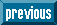## Indicators

•Cited by SciELO
•Access statistics

•Similars in SciELO

## Print version ISSN 0035-001X

### Rev. mex. fis. vol.65 n.6 México Nov./Dec. 2019  Epub May 30, 2020

#### https://doi.org/10.31349/revmexfis.65.690

Research

in Solid State Physics

Preparation, optical characterization and stability of gold nanoparticles by facile methods

aUniversidad Autónoma de Nuevo León, Facultad de Ciencias Químicas, Pedro de Alba S/N, Ciudad Universitaria, 66450 San Nicolás de Los Garza, Nuevo León, México.

Abstract

Gold nanoparticles were obtained by reduction of chloroauric acid HAuCl4 by two methods: conventional heating and microwave synthesis. In the microwave method, different cycles and sonication time were analyzed to establish the synthesis with smaller nanoparticle size and higher absorbance. The influence of synthesis method, cycles quantity and sonication time in the gold nanoparticles size and plasmon resonance were studied. Gold nanoparticles were analyzed by UV-Vis spectrophotometry, dynamic light scattering and surface electron microscopy. The results indicate that the nanoparticles obtained by microwave have bigger size than those obtained by conventional heating, however, present higher absorption. The microwave nanoparticles were analyzed one year after its synthesis and it was found that the plasmon resonance signal remains almost unchanged. Finally, two equations were derived from the analyzes for estimate molar extinction coefficient, molar concentration, and nanoparticle average diameter.

Keywords: Synthesis; stability; gold nanoparticles

PACS: 32.30.Jc; 61.46.+w; 71.45.Gm; 81.20.-n

1. Introduction

In recent years, gold nanoparticles have become the object of numerous studies due to their unique optical and physical properties. These nanoparticles have an endless number of applications in medicine [1,2], sensors [3,4], nanoengineering , solar cells [6,7], among others [8-11]. All these areas exploit the optical property of Surface Plasmon Resonance (SPR) which oscillates around 500 nm depending on the size of the nanoparticle.

A great variety of syntheses have been proposed over the years, being the Chloroauric acid HAuCl4 the most used precursor for colloidal gold. These variations include the use of reducing agents , stabilizing agents , reaction medium ,pH control , reaction temperature , among others. Gold nanoparticles are obtained mainly by a conventional heating method and microwave synthesis , being the latter the most studied in recent years, due to short synthesis times and small amounts of reagent. The synthesis conditions influence the particle size and therefore the SPR.

Gold nanoparticles are mainly characterized by UV-Vis absorption spectrum, with which the extinction coefficient can be calculated if other parameters are known. Extinction coefficient (ε) is an important parameter that allows estimating the average diameter and the nanoparticles concentration; however, it is necessary to know the molar concentration, which is a problem due to the low monodispersity of the nanoparticles. Here are presented theoretical calculations of this coefficient to show its dependence with the size of the nanoparticles.

In this article, conventional heating, microwave synthesis methods, as well as the influence of sonication and microwave cycles on the size of the nanoparticle and the effect on the SPR were studied. Besides, spectrophotometry UV-Vis and scanning electron microscopy (SEM) analysis were performed on the nanoparticles obtained by microwave one year after its synthesis. Two equations were established to estimate the average diameter and the molar concentration of the solution.

2. Experimental

The gold nanoparticles presented in this research were obtained from HAuCl4, using sodium citrate as a reducing and stabilizing agent. These nanoparticles were synthesized by thermal and microwave heating methods to observe the difference of the size and plasmon signal obtained by both methods. The details of the syntheses are presented below.

2.1. Thermal heating method

For the synthesis of gold nanoparticles, 1 mL was taken from a solution of HAuCl4 25 mM and was gauged to 100 mL. This solution was let under string and heating until boil. After, 5 mL of 1 wt% sodium citrate was added; the solution, originally yellow, turned colorless and finally changed to red wine. The solution was let boil for 30 minutes and finally was gauged to 100 mL to compensate the evaporation losses.

2.2. Microwave method

For the microwave synthesis, 1 mL of HAuCl4 5 mM and 1 mL of sodium citrate 25 mM were dissolved in 18 mL of H2O. This solution was placed into a conventional microwave Whirlpool® model WM1207D, for 10 min at a power of 1275W to 20%. The result was a purple solution.

To study the effect on the size and plasmonic signal, two variables were studied in this method: cycles and sonication time. Cycles were varied in 0, 5 and 10, while the sonication time was 10, 15 and 20 min.

3. Results and Discussion

Colloidal gold nanoparticles interact with the light according to its size and environment. Depending on its dimensions, the solutions can be blue, yellow, red, purple, among other colors. The origin of these colors is attributed to the collective oscillation of the conduction electron in the surface of the metal; a phenomenon known as SPR. Mie was the first to describe these oscillations quantitatively through the resolution of Maxwell's Equations. The Mie theory allows interpreting experimental results .

3.1. UV-Vis characterization

3.1.1. Thermal heating method

Gold nanoparticles synthesized under conventional heating method present the characteristic SPR (around 526 nm), however, this synthesis presents low absorbance as can be observed in Fig. 1.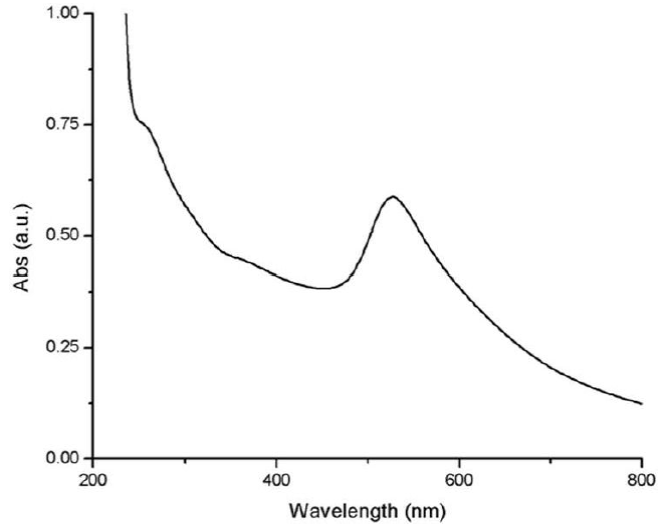Figure 1 The absorption spectrum of gold NP synthesized by the conventional heating method.

The Lambert-Beer law indicates that the absorbance is directly proportional to the extinction coefficient multiplied by the path length and the concentration of the solution. Therefore, lower absorption it's related to a low concentration of gold nanoparticles. On the other hand, according to Mie, the oscillation modes depend on the particle size and as the size decreases, the maximum absorption decreases [19,20] and shifts to the blue [21-24]. As the size of the nanoparticles increases, the mean free path of the electrons is enhanced, therefore, an increase in absorbance is expected [25-27].

3.1.2. Microwave

Microwave radiation can penetrate the reaction solution with different wavelength (than thermal heating) to heating the solution uniformly, so the concentration of gold nanoparticles is expected to be higher than thermal heating. According to this, it was decided to analyze different conditions of synthesis.

Without cycles

All the nanoparticles obtained by microwave synthesis for 10 min without cycles, present the characteristic SPR (around 535). In Fig. 2 it is appreciated that these nanoparticles show higher absorption than the obtained by the thermal heating method. Also, its maximum wavelength is shifted to the red, as was expected because the purple color indicates approximate sizes of 50 nm, while the red color obtained in the synthesis of conventional heating indicates sizes around 5nm .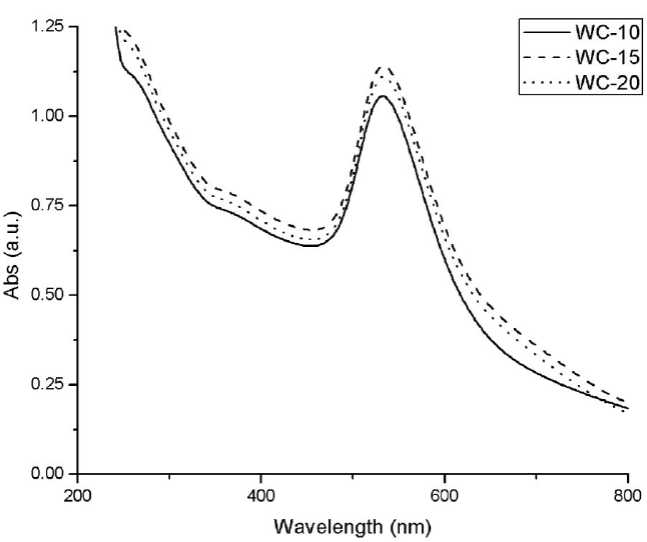Figure 2 The absorption spectrum of gold nanoparticles synthesized by microwave without cycles.

5 cycles

Gold nanoparticles obtained by microwave synthesis in 5 cycles show also the SPR. 5C-20 synthesis shows the highest absorbance indicating, a higher concentration of gold nanoparticles (Fig. 3). A greater number of nanoparticles increases the total surface for SPR and, as a consequence, an increase in absorption is observed [29,30].Figure 3 The absorption spectrum of gold nanoparticles synthesized by microwave in 5 cycles.

10 cycles

Gold nanoparticles obtained by microwave synthesis in 10 cycles show the SPR. However, it can be observed in Fig. 6 that, for the 10C-15 synthesis, the absorbance is as low as the nanoparticles obtained by the thermal heating method, possibly for the low stability that is obtained under this synthesis, as mentioned earlier (Fig. 4).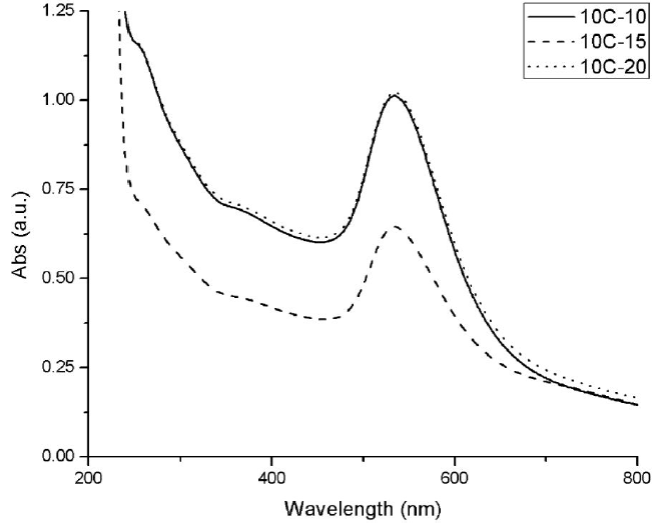Figure 4 The absorption spectrum of gold nanoparticles synthesized by microwave in 10 cycles.

A higher concentration of gold nanoparticles is obtained under microwave radiation; remembering that thermal heating method has a greater concentration of sodium citrate, absorbance is expected to be lower.

In a joint analysis for the two techniques (dispersion light scanning (DLS) and UV-Vis Spectrometry), it can be conclude that the ideal gold nanoparticles are those obtained by synthesis in microwave for 10 minutes without cycles and sonication of 20 minutes, because they present the higher absorption and smaller distribution of size, compared with the rest of the synthesis, being both important parameters to consider in optoelectronic applications.

3.2. Dynamic light scattering

3.2.1. Thermal heating method

Gold nanoparticles obtained under conventional heating method, have a size between 5 nm and 10 nm, showing narrow size distribution as can be observed in Fig. 5.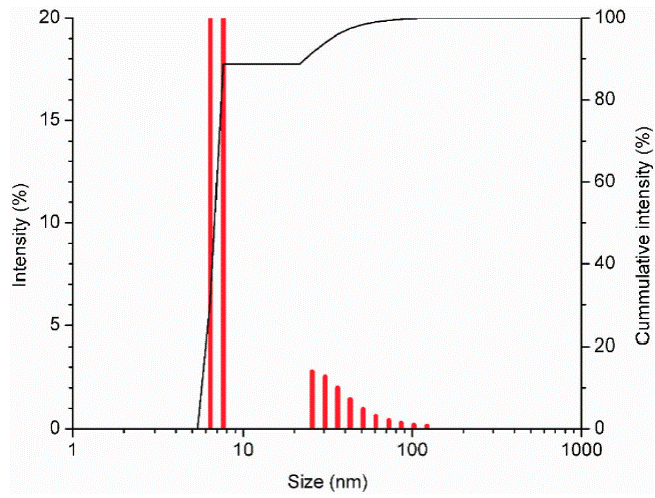Figure 5 DLS analysis of gold NP obtained by the conventional heating method.

3.2.2. Microwave method Without cycles

In microwave synthesis for 10 min without cycles, sonication times were varied in 10 min (WC-10), 15 min (WC-15) and 20 min (WC-20). According to the results, increasing sonication time, the size and size distribution of the nanoparticles are reduced. See Fig. 6.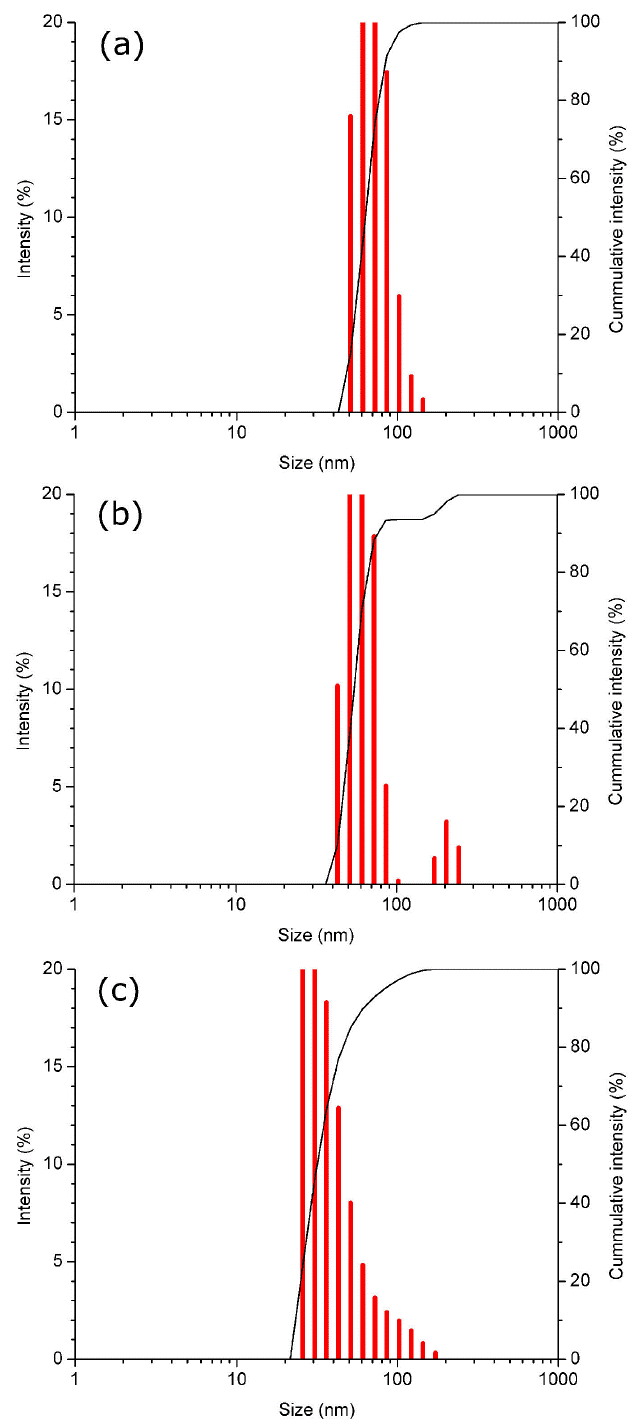Figure 6 DLS analysis of gold nanoparticles synthesized by microwave without a cycle, with a variation of the sonication time in 10, 15 and 20 min.

5 cycles

In synthesis for 10 min with 5 cycles (2 min on, 30 secs off), sonication times were also varied in 10 min (5C-10), 15 min (5C-15) and 20 min (5C-20). Once again, it can be observed how increasing sonication time, the size and size distribution of the nanoparticle (NP) are reduced (Fig. 7). However, the size distribution is bigger than the synthesis without cycles because maintaining a uniform heating temperature is a critical parameter to achieve a narrow size distribution .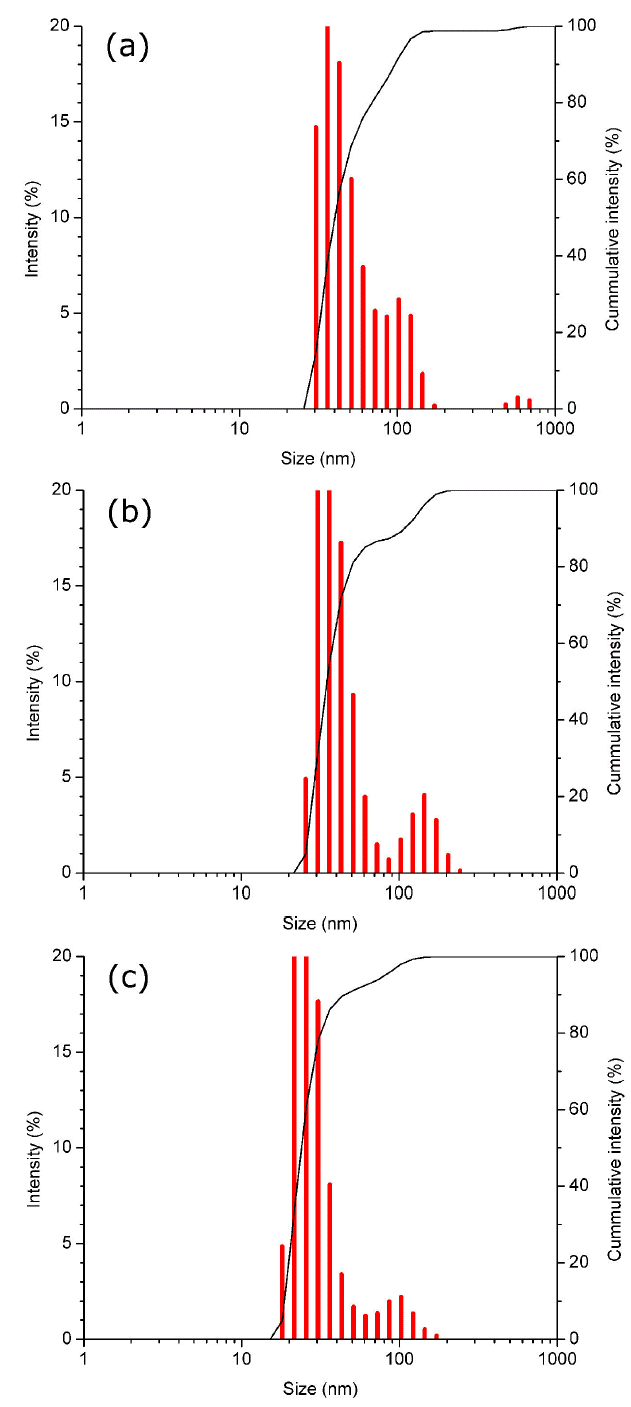Figure 7 DLS analysis of gold nanoparticles synthesized by microwave in 5 cycles, with a variation of the sonication time in 10, 15 and 20 min.

10 cycles

In synthesis for 10 min with 10 cycles (1 min on, 30 secs off), sonication times were also varied in 10 min (10C-10), 15 min (10C-15) and 20 min (10C-20). However, under these conditions, the synthesis showing the smallest nanoparticle size is 10C-10 follow by 10C-20 and finally 10C-15. This probably because nanoparticles synthesized under 10 cycles show less stability than the synthesized under 5 cycles and no-cycles, due to the heating is interrupted by cycles resulting in a wider distribution of size, as mentioned before. See Fig. 8.Figure 8 DLS analysis of gold nanoparticles synthesized by microwave in 10 cycles, with a variation of the sonication time in 10, 15 and 20 min.

According to the DLS analysis, the gold nanoparticle size is reduced as cycles increase. This can be explained by the reaction times. Despite all the synthesis are of 10 minutes in microwave, the time in which the solution stays warm increases with the cycles, i.e. in the synthesis of 5 cycles (2 min on and 30 sec off), the total reaction time is 12 min since in the 30 seconds off, the solution does not reach room temperature; in the synthesis of 10 cycles (1 min on and 30 sec off), the total reaction time is 14 min. As the time of the reaction increases, sodium citrate continues stabilizing and reducing gold nanoparticles.

Comparing the results obtained by conventional thermal heating method and microwave heating, it can be observed that the first one presents a narrow size distribution, even though the heating by microwave radiation is more uniform, therefore, the synthesis of thermal heating is about 30-40 min which allows reducing the volume of the solution, reducing, in turn, the temperature gradient, making heating more uniform. Also, it has been known that increasing the concentration of sodium citrate the size distribution becomes narrower .

3.3. Stability

3.3.1. Stability of plasmon resonance

An additional analysis was made, to observe the stability of the plasmon resonance signal of the nanoparticle solution. The synthesis in the microwave without cycles and 20 min of sonication was let in a dry and dark ambient and its UV-Vis spectrum was measured exactly one year later after its synthesis. As can be observed in Fig. 9, the plasmon signal presents a decrease almost null, which is indicative that the nanoparticles remained without agglomerating with each other. Avoid agglomeration with the passage of time is an important factor for the nanoparticles synthesis because as the size of the nanoparticle increases, the plasmon resonance signal decrease and therefore the optical properties are lost. It is important to mention that the nanoparticles obtained were let in its reaction medium, which could have helped to maintain its stability.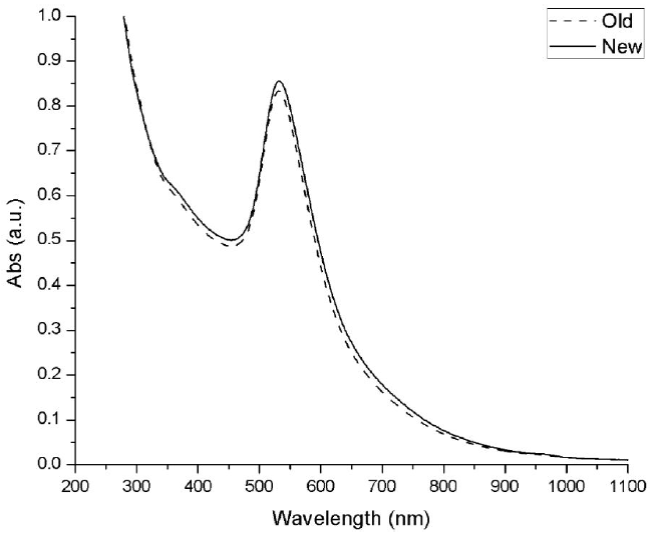Figure 9 The absorption spectrum of gold nanoparticles synthesized by microwave without cycles. The solid line represents the newly synthesized nanoparticles and dash line represents the same nanoparticle solution one year after its synthesis.

3.3.2. Stabilityofsize nanoparticle

One of the main problems in nanoparticles solutions is the tendency to agglomerate with the passage of time, having as a consequence an increase in size and the loss of properties. Despite the stability of plasmon resonance, the nanoparticle size tends to increase with time.

Gold nanoparticles (and in general all the nanoparticles) tends to form agglomerates, that can be reduced by sonica-tion. Figure 10 shows a comparison of the newly synthesized nanoparticles and after one year. Images labeled as (a) and (b) belong to the "fresh synthesis" in which can be appreciated the nanospheres in an isolated way; (c) and (d) shows the agglomerated formed by these nanoparticles after one year of its synthesis.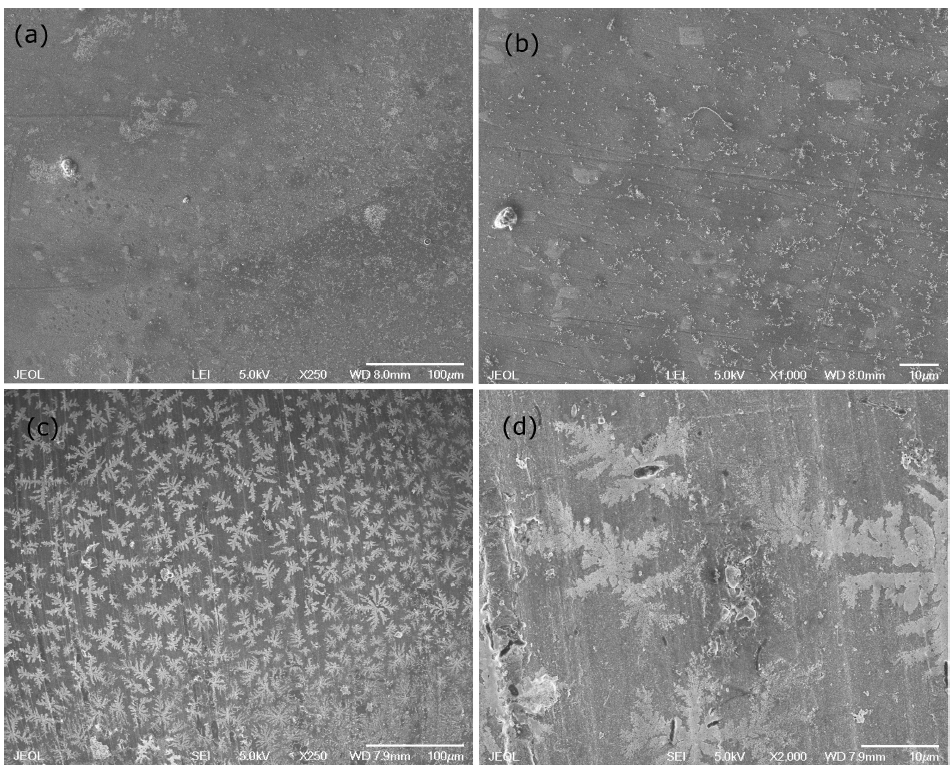Figure 10 Surface electron microscopy images of gold nanoparticles synthesized by microwave. (a) and (b) are the newly synthesized nanoparticles; (c) and (d) are the nanoparticles after one year.

According to measurements made in an image analyzer, the newly synthesized nanoparticles have an average size between 20 nm and 30 nm (Fig. 11a), which can be increased up to 70 nm after one year of being synthesized (Fig. 11b).Figure 11 Surface electron microscopy of gold nanoparticles comparing size: (a) newly synthesized and (b) after one year.

In addition to the size increase, these nanoparticles tend to modify its original form by joining each other to form more complex forms. Figure 12 presents a micrography where, besides the nanospheres, nanorods, and nanotriangles of gold are clearly observed.Figure 12 Micrograph of gold nanoparticles after one year of its synthesis, showing different nanoforms.

3.4. Extinction coefficient

The extinction coefficient is a measure of how strongly a species absorbs light at a given wavelength. It can be calculated by the Lambert-Beer law, however, it is necessary to know the molar concentration, which is actually a challenge, as was mentioned in the introduction. Below is shown a simple method for obtaining the extinction coefficient.

3.4.1. Gold atoms per nanoparticle

The average number of gold atoms was calculated by Eq. (1). For calculating the average number of gold atoms contained in a nanoparticle, a spherical shape was assumed. Eq. (1) was used for this estimation, where ρ is the density of gold (1.93 x 10-20 g/nm3), Na is the Avogadro constant (6.02214179 x 1023), M is the atomic mass of gold (197 g/mol) and D is the average diameter of nanoparticles in nm .

3.4.2. Molar concentration

Equation 2 was used for the determination of the molar concentration of the solutions, where N Total is the number of gold atoms in the initial solution, V is the reaction volume and N was obtained from the previous equation.

N=NTotalN V NA (2)

3.4.3. Estimation of the coefficients

Extinction coefficients (ε) of gold nanoparticles were calculated according to Lambert-Beer law Eq. (3), where A represents the absorbance in a specific wavelength, l is the path length in cm and C is the molar concentration of the solution.

A=εlC (3)

Table I summarizes the molecular concentration and extinction coefficients calculated for each synthesis. It is evident how the extinction coefficient increased, in order of magnitude, with the average size of nanoparticle [33-36]. This behavior can be explained as follow: an increase in the diameter of the nanoparticle, leads to a decrease in the concentration, therefore an increase in the extinction coefficient is obtained, as can be appreciated in Eqs. (1), (2) and (3). Results are according to the reported by El-Sayed .

Table I Extinction coefficients (ε) of the different synthesized nanoparticles.

 Synthesis Average D (nm) Concentration (mol/L) Maximum wavelength ε(M-1cm-1 ) WC-10 65 2.94644E-11 533 1.81E+13 WC-15 55 4.86351E-11 534 1.10E+13 WC-20 27 4.11099E-10 534 1.30E+12 5C-10 38 1.47464E-10 534 3.62E+12 5C-15 35 1.88727E-10 534 2.83E+12 5C-20 22 7.59923E-10 535 7.04E+11 10C-10 28 3.68607E-10 534 1.45E+12 10C-15 40 1.26432E-10 535 4.23E+12 10C-20 30 2.99691E-10 534 1.78E+12

This dependence is also reported by the Drude model, in which the relaxation or damping frequency is related to the mean free path of the conduction electron and can be calculated as follow:

ωd=vfRbulk. (4)

Where ω d is the damping frequency, v f the velocity of electrons at the Fermi energy and Rbulk is mean free path of the conduction electrons.

In the case of the nanoparticle, radio is smaller than the mean free path, this parameter becomes size dependent due to the additional scattering of the electrons caused by the surface:

1Reff=1R+1Rbulk. (5)

Where R eff is the mean free path and R is the particle radio.

From Eqs. (4) and (5) it can be appreciated the dependence of the particle size; a decrease in the particle size leads to an increase in damping frequency, causing the maximum absorption intensity to decrease .

The extinction coefficients were calculated at the maximum absorbance wavelength, i.e. plasmon signal. The variation of the extinction coefficients according to the molar concentration of the gold nanoparticles synthesized by microwave is presented in Fig. 13 showing an exponential behavior.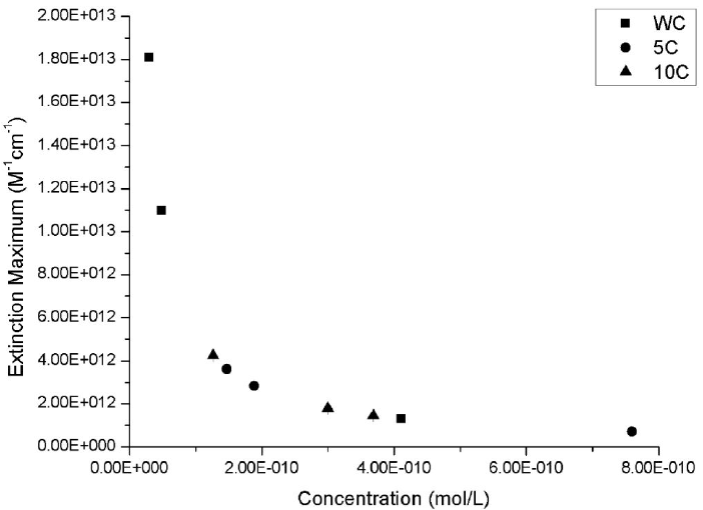Figure 13 Extinction coefficients of gold nanoparticle synthesized by microwave. Squares represent the gold nanoparticles obtained in continuous heating (without cycles), circles represent the synthesis in 5 cycles and triangles represent the synthesis in 10 cycles.

The graph behavior was adjusted to a potential type trend line, getting Eq. (4) with a coefficient of determination (R2) of 0.99999.

ε=542.61*C-0.999 (6)

The natural logarithm of the extinction coefficient was also plotted against the natural logarithm of the diameter of the nanoparticles (Fig. 14). A linear adjustment was made, obtaining a coefficient of determination (R 2) of 0.99999 in accordance with Mie theory [39,40]. The Equation obtained is presented in Eq. (5).

In(ε) = 2.99782 * In(D) + 18.0126. (7)Figure 14 The linear fitting curve of the natural logarithm of the extinction coefficients obtained for the different synthesis vs natural logarithm of the diameter of gold nanoparticles synthesized.

Equations (4) and (5) can be used to estimate important parameters of a gold nanoparticle solution such as average diameter, molar concentration, and even the extinction coefficient, as long as one of the parameters has been obtained by a characterization analysis. Finding the maximum absorption wavelength, the solution molar concentration can be obtained. On the other hand, if the average diameter is known, the extinction coefficient can be estimated. This can be useful for experiment planning without the need for extensive characterization.

4. Conclusions

The gold nanoparticles present in this paper were synthesized by thermal heating and microwave methods. Comparing these two techniques it is concluded that the smallest nanoparticles are obtained by conventional heating, however, present low absorbance which indicates a low concentration. In the microwave method, the influence of sonication time and cycles on nanoparticle size and plasmon resonance was observed, finding that a synthesis without cycles and 20 min of sonication gave nanoparticles with narrow size distribution and higher plasmon resonance, i.e. a more homogenous distribution of sizes and a higher concentration is obtained. These nanoparticles increase its size after one year, nevertheless plasmon resonance remains almost unaffected. Besides, two equations were formulated to estimate the nanoparticle average diameter and the molar concentration of a solution by a simple method.

Acknowledgments

This work was financially supported by CONACYT under the project PN-150, and the Universidad Autónoma de Nuevo León.

References

1. J. Valdez, S. Bawage, I. Gomez, S. R. Singh, J. Nanobiotechnology 14 (2016) 13. [ Links ]

2. M. Sengani, A. M. Grumezescu, V. D. Rajeswari, Open Nano 2 (2017) 37. [ Links ]

3. L. Fritea et al ., J. Electroanal. Chem. (2018). [ Links ]

4. H. Chen, K. Zhou, G. Zhao, Trends Food Sci. Technol. 78 (2018) 83. [ Links ]

5. V. Gonzalez, C. Puente, I. Lopez. Handb, Nanomater. Ind. Appl. 1012 (2018). [ Links ]

6. J. Hao et al ., Opt. Commun. 362 (2016) 50. [ Links ]

7. Y. H. Lee, D. H. Kim, T. W. Kim, Org. Electron. 37 (2016) 74. [ Links ]

8. J. Luo, J. Chen, H. Wang, H. Liu, J. Power Sources 303 (2016) 287. [ Links ]

9. A. A. Borran, A. Aghanejad, A. Farajollahi, J. Barar, Y. Omidi, Radiat. Phys. Chem. 152 (2018) 137. [ Links ]

10. I. Dahan, S. Sorrentino, R. Boujemaa-Paterski, O. Medalia. Structure 26 (2018) 1408. [ Links ]

11. A. Staykov, T. Miwa, K. Yoshizawa, J. Catal. 364 (2018) 141. [ Links ]

12. K. Zhang et al ., Carbohydr. Polym. 186 (2018) 132. [ Links ]

13. R. Zarzuela et al ., J . Photochem. Photobiol. B Biol. 179 (2018) 32. [ Links ]

14. M. Hamelian, S. Hemmati, K. Varmira, H. Veisi, J. Taiwan Inst. Chem. Eng. (2018). [ Links ]

15. W.-Y. Qiu et al ., Int. J. Biol. Macromol. 106 (2018) 498. [ Links ]

16. N. P. B. Tan, C. H. Lee, P. Li, Data Br. 5 (2015) 434. [ Links ]

17. E. Ferrer et al ., Mater. Res. Bull. 47 (2012) 3835. [ Links ]

18. S. Link, M. A. El-Sayed, J. Phys. Chem. B 103 (1999) 4212. [ Links ]

19. Y. H. Su et al ., Nanoscale 2 (2010) 2639. [ Links ]

20. R. Tsekov, P. Georgiev, S. Simeonova, K. Balashev, Comptes rendus l'Académie Bulg. des Sci. Sci. mathématiques Nat. 70 (2017) 1237. [ Links ]

21. S. Muhlig et al ., Optics express 19 (2011). [ Links ]

22. D. M. P. Mingos. Gold Clusters, Colloids and Nanoparticles I 161, (Springer International Publishing, 2014). [ Links ]

23. X. Huang, M. A. El-Sayed, J. Adv. Res. 1 (2010) 13. [ Links ]

24. T. Nakamura et al ., AIP Adv. 3 (2013) 82101. [ Links ]

25. A. Zuber et al ., Sensors Actuators B Chem. 227 (2016) 117. [ Links ]

26. W. Haiss, N. T. K. Thanh, J. Aveyard, D. G. Fernig, Anal. Chem. 79 (2007) 4215. [ Links ]

27. M. Novakovic et al ., Appl. Surf. Sci. 426 (2017) 667. [ Links ]

28. Z. P. Aguilar, in Nanomaterials for Medical Applications (ed. Aguilar, Z. P. B. T.-N. for M. A.) 33, (Elsevier, 2013). [ Links ]

29. M. Abdelhalim, M. Mady, M. Ghannam, Nanomedicine and Nanotechnology 03 (2012). [ Links ]

30. Y.-H. Su, Y.-F. Ke, S.-L. Cai, Q.-Y. Yao, Light Sci. and Amp; Appl. 1 (2012) e14. [ Links ]

31. N. V. K. Thanh, N. D. Giang, L. Q. Vinh, H. T. Dat, Commun. Phys. 24 (2014) 146. [ Links ]

32. X. Liu, M. Atwater, J. Wang, Q. Huo, Colloids Surfaces B Biointerfaces 58 (2007) 3. [ Links ]

33. P. Georgiev et al. Colloids Surfaces A Physicochem. Eng. Asp. 434 (2013) 154. [ Links ]

34. P. Georgiev et al. Colloids Surfaces A Physicochem. Eng. Asp. 494 (2016) 39. [ Links ]

35. L. Liz-Marzan, Materials Today 7 (2004). [ Links ]

36. K. Kolwas, A. Derkachova, M. Shopa, J. Quant. Spectrosc. Radiat. Transf. 110 (2009) 1490. [ Links ]

37. P. K. Jain, K. S. Lee, I. H. El-Sayed, M. A. El-Sayed, J. Phys. Chem. B 110 (2006) 7238. [ Links ]

38. P. Mulvaney, Langmuir 12 (1996) 788. [ Links ]

39. H. Horvath, J. Quant. Spectrosc. Radiat. Transf. 110 (2009) 783. [ Links ]

40. G. Baffou, http://www.Guillaume.Baffou.Com/Courses.Php 1 (2017). [ Links ]

Received: March 13, 2019; Accepted: May 14, 2019This is an open-access article distributed under the terms of the Creative Commons Attribution License